#Units of Conversion: Linear MeasureBasic to the understanding of unit conversion is the understanding equivalence, the understanding of the multiplicative identity of 1, and how the two are related. For a review of these concepts, click on Review.

If you have forgotten how to manipulate fractions, visit Fractions for Adults on SOSMath for an in-depth review.

Review the following table (Webster's New World Dictionary, Second Edition, Simon and Schuster, 1982) of Standard Units of Conversion to see if you can create fractions that have a value of 1. You can click to examples following each table.

LinearMeasure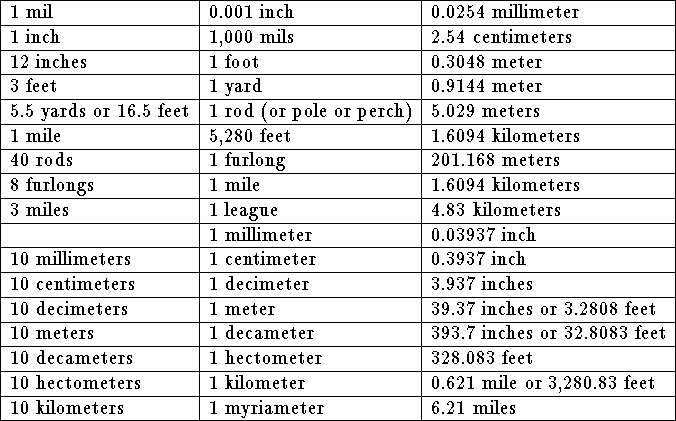Example 1. Convert 4.6 miles to feet.

Solution.

• Write 4.6 miles as a fraction by dividing it by 1: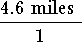.

• Multiply the fraction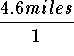by 1: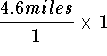• Note: We know that the fraction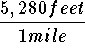is equivalent to 1 because the numerator and the denominator measure the same length.

• Convert the number 1 in the expressionto the equivalent fractionso that the expressioncan be written as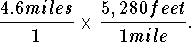• Rewrite 4.6 miles as 4.6 x 1 mile and 5280 feet as 5280 x 1 foot, and rewrite the two fractions as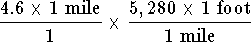• Multiply the two fractions: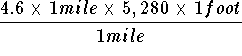• Rearrange the numerator: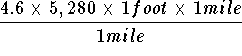• Write the above fraction as two fractions: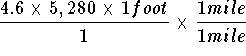• Replace the fraction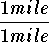by 1: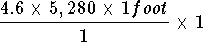• Simplify: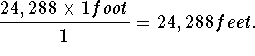If you would like to review another example, click on Example.

If you would like to practice some problems click on Problems.[Units of Conversion]S.O.S MATHematics home page

Do you need more help? Please post your question on our S.O.S. Mathematics CyberBoard.Author: Nancy Marcus